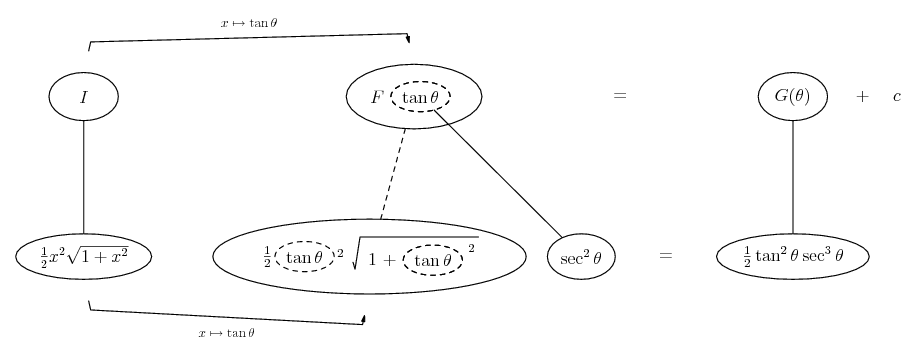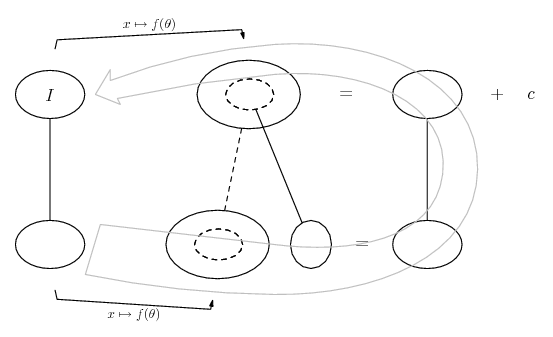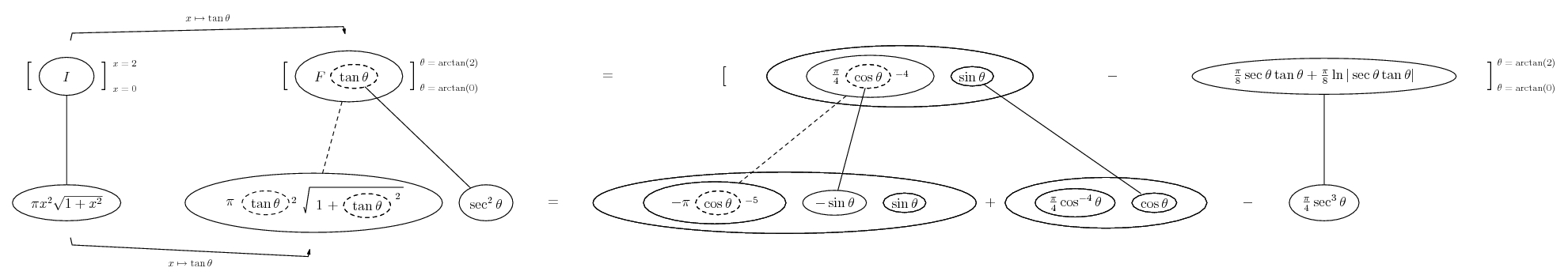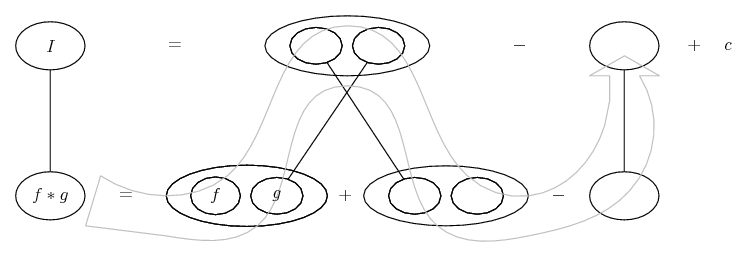# Thread: Surface area of revolution [another question]

1. ## Surface area of revolution [another question]

The curve $\displaystyle C$ has equation

$\displaystyle y =\frac{1}{2}x^2$.

a] use the substitution $\displaystyle x=sinhu$ to evaluate the arc length of $\displaystyle C$ correct to 2 decimal places.

b] The arc of $\displaystyle C$ joining the points $\displaystyle (0,0)$ and $\displaystyle (2,2)$ is rotated through four right angles about the x-axis. Find the area of the curved surface generated correct to 2 decimal places.

I've done part a] - got 2.96.

Part b] is giving me significant trouble though. I write the integral as

$\displaystyle 2\pi \int_0^2 \frac{1}{2}x^2 \sqrt{1+x^2} dx$ and from here, I've tried a variety of techniques - I made the $\displaystyle x^2$ term into $\displaystyle \sqrt{x^4}$ and used the rule $\displaystyle \sqrt{a} . \sqrt{b} = \sqrt{ab}$ but the answer was wrong, and I tried the same hyperbolic substitution, which also gave me a wrong answer. I know this because the answer is written down on a sheet with no working to show it was obtained. Please could you suggest a suitable way to approach the question? Any help is greatly appreciated2. x = tan t and then you're basically doing

http://www.mathhelpforum.com/math-he...egral-5-a.html

3.Originally Posted by tom@ballooncalculusx = tan t and then you're basically doing

http://www.mathhelpforum.com/math-he...egral-5-a.html
How did you figure out that x=tan t should be used? I've had a look at a possible evaluation of the integral, given this substitution and it looks horrifying, as does what is in the quoted link.

4. Trigonometric substitution - Wikipedia, the free encyclopedia

gives the strategies. Pic on its way.

Edit:

Just in case a picture helps...... where... is the chain rule. Straight continuous lines differentiate downwards (integrate up) with respect to x, and the straight dashed line similarly but with respect to the dashed balloon expression (the inner function of the composite which is subject to the chain rule).

The general drift is...And the rest...

Spoiler:...where... is the drift for the parts process, except we've zoomed in on the embedded chain rule. For $\displaystyle \int \sec^3 \theta\ d \theta$ see http://en.wikipedia.org/wiki/Integral_of_secant_cubed

Plug in the limits and use a triangle diagram or http://staff.jccc.net/swilson/trig/compositions.htm to simplify the trig.
________________________________________

Don't integrate - balloontegrate!

Balloon Calculus; standard integrals, derivatives and methods

Balloon Calculus Drawing with LaTeX and Asymptote!

#### Search Tags

area, question, revolution, surface